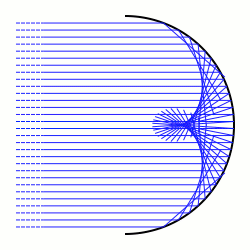# Caustic (mathematics)

For other uses, see Caustic (disambiguation).In differential geometry and geometric optics, a caustic is the envelope of rays either reflected or refracted by a manifold. It is related to the concept of caustics in optics. The ray's source may be a point (called the radiant) or parallel rays from a point at infinity, in which case a direction vector of the rays must be specified.

More generally, especially as applied to symplectic geometry and singularity theory, a caustic is the critical value set of a Lagrangian mapping (πi) : LMB; where i : LM is a Lagrangian immersion of a Lagrangian submanifold L into a symplectic manifold M, and π : MB is a Lagrangian fibration of the symplectic manifold M. The caustic is a subset of the Lagrangian fibration's base space B.

## Catacaustic

A catacaustic is the reflective case.

With a radiant, it is the evolute of the orthotomic of the radiant.

The planar, parallel-source-rays case: suppose the direction vector isand the mirror curve is parametrised as. The normal vector at a point is; the reflection of the direction vector is (normal needs special normalization)Having components of found reflected vector treat it as a tangentUsing the simplest envelope formwhich may be unaesthetic, butgives a linear system inand so it is elementary to obtain a parametrisation of the catacaustic. Cramer's rule would serve.

### Example

Let the direction vector be (0,1) and the mirror beThenandhas solution; i.e., light entering a parabolic mirror parallel to its axis is reflected through the focus.

## References

1. Arnold, V. I.; Varchenko, A. N.; Gusein-Zade, S. M. (1985). The Classification of Critical Points, Caustics and Wave Fronts: Singularities of Differentiable Maps, Vol 1. Birkhäuser. ISBN 0-8176-3187-9.